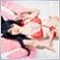# EA.mq4 Array of Range Error11

I need help with this code I can't find any solution.

Array Out Range on Part

```breakLow[x]=0.0;
breakHi[x]=0.0;```

I tried to use ArrayInitialize and it seems it solve the problem in that part.

BUT

next problem appeared

on

```      if(hi[x]>0 || low[x]>0)//<------------------- Array OUT OF RANGE HERE
{
for(i=x;i<=x+20;i++)
{// cari cbhi/cbLow
if(cbHi[i]>0)
{
cbhi=cbHi[i];
break;
}
else cbhi=0;

if(cbLow[i]>0)
{
cblow=cbLow[i];
break;
}
else cblow=0;
}
}
```
```   //ERROR ARRAY OUT OF RANGE MAIN CODE

double cbHi[],cbLow[],hi[],low[],breakHi[],breakLow[];
double cbhi,cblow;
cbhi=cblow=0;
int x,i,y;
double highest,lowest;
datetime T1,T2;
for(x=0;x<=Limit;x++)
{
breakLow[x]=0.0;
breakHi[x]=0.0;
if(hi[x]>0 || low[x]>0)
{
for(i=x;i<=x+20;i++)
{
if(cbHi[i]>0)
{
cbhi=cbHi[i];
break;
}
else cbhi=0;

if(cbLow[i]>0)
{
cblow=cbLow[i];
break;
}
else cblow=0;
}
}
for(y=x;y>=0;y--)
{// cari break;
if(cbhi>0 || cblow>0)
{
if(iClose(Symbol(),0,y)>cbhi && cbhi>0 && low[x]>0)
{
breakHi[y]=iClose(Symbol(),0,y);
cbhi=0;
highest=breakHi[y];
lowest=iLow(Symbol(),0,x);
T1=iTime(Symbol(),0,x);
T2=iTime(Symbol(),0,x+2);
break;
}
else breakHi[y]=0.0;

if(iClose(Symbol(),0,y)<cblow && cblow>0 && hi[x]>0)
{
breakLow[y]=iClose(Symbol(),0,y);
cblow=0;
highest=breakLow[y];
lowest=iHigh(Symbol(),0,x);
T1=iTime(Symbol(),0,x);
T2=iTime(Symbol(),0,x+2);
break;
}
else breakLow[y]=0.0;
}
}

}
```20214

`double cbHi[],cbLow[],hi[],low[],breakHi[],breakLow[];`
All those arrays have no size, so any access is a range error. Perhaps you want to make them indicator buffers.